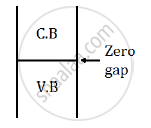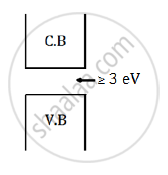# Distinguish Between a Conductor and a Semi Conductor on the Basis of Energy Band Diagram - Physics

Distinguish between a conductor and a semi conductor on the basis of energy band diagram

#### Solution

Distinction between conductor and insulator:

Conductor: In a conductor, the valance band is either partially filled or completely filled.

It has zero forbidden energy gap between the conduction band and the valance band.

The energy band diagram can be represented as shown below:Insulator: In an insulator, the valance band is completely filled and the conduction band is empty.

The forbidden energy gap between the conduction band and the valance band is greater than or equal to 3 eV

The energy band diagram can be represented as shown below:Concept: Energy Bands in Conductors, Semiconductors and Insulators
Is there an error in this question or solution?